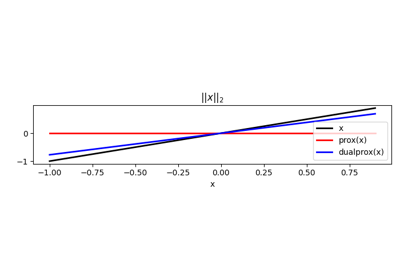# pyproximal.Euclidean#

class pyproximal.Euclidean(sigma=1.0)[source]#

Euclidean norm proximal operator.

Proximal operator of the Euclidean norm: $$\sigma \|\mathbf{x}\|_2 = \sigma \sqrt{\sum x_i^2}$$.

Parameters
sigmaint, optional

Multiplicative coefficient of $$L_{2}$$ norm

Notes

The Euclidean proximal operator is defined as:

$\prox_{\tau \sigma \|\cdot\|_2}(\mathbf{x}) = \left(1 - \frac{\tau \sigma }{\max\{\|\mathbf{x}\|_2, \tau \sigma \}}\right) \mathbf{x}$

This operator is sometimes called block soft thresholding.

Moreover, as the conjugate of the Euclidean norm is the orthogonal projection of its dual norm (i.e., Euclidean norm) onto a unit ball, its dual operator is defined as:

$\prox^*_{\tau \sigma \|\cdot\|_2}(\mathbf{x}) = \frac{\sigma \mathbf{x}}{\max\{\|\mathbf{x}\|_2, \sigma\}}$

Methods

 __init__([sigma]) affine_addition(v) Affine addition chain(g) Chain grad(x) Compute gradient postcomposition(sigma) Postcomposition precomposition(a, b) Precomposition prox(**kwargs) proxdual(**kwargs)

## Examples using pyproximal.Euclidean#Norms

Norms